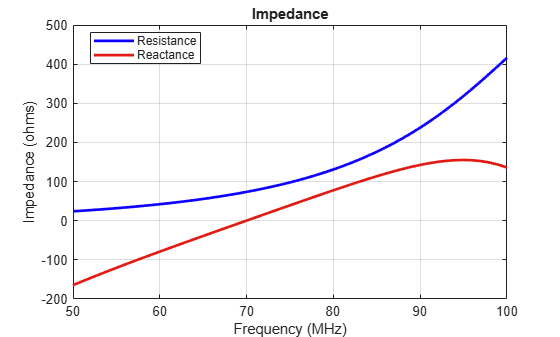# impedance

Input impedance of antenna; scan impedance of array

## Syntax

``impedance(antenna,frequency)``
``z = impedance(antenna,frequency)``
``impedance(array,frequency,elementnumber)``
``z = impedance(array,frequency,elementnumber)``

## Description

example

````impedance(antenna,frequency)` calculates the input impedance of an antenna object and plots the resistance and reactance over a specified frequency. ```

example

````z = impedance(antenna,frequency)` returns the impedance of the antenna object, over a specified frequency.```
````impedance(array,frequency,elementnumber)` calculates and plots the scan impedance of a specified antenna element in an array.```
````z = impedance(array,frequency,elementnumber)` returns the scan impedance of a specified antenna element in an array. ```

## Examples

collapse all

Calculate and plot the impedance of a planar dipole antenna over a frequency range of 50MHz - 100MHz.

```h = dipole; impedance (h,50e6:1e6:100e6);```Calculate scan impedance of default linear array over a frequency range of 50MHz to 100MHz.

```h = linearArray; z = impedance(h,50e6:1e6:100e6)```
```z = 51×2 complex 102 × 0.2976 - 1.7632i 0.2976 - 1.7632i 0.3089 - 1.6801i 0.3089 - 1.6801i 0.3204 - 1.5989i 0.3204 - 1.5989i 0.3322 - 1.5193i 0.3322 - 1.5193i 0.3441 - 1.4411i 0.3441 - 1.4411i 0.3564 - 1.3643i 0.3564 - 1.3643i 0.3689 - 1.2887i 0.3689 - 1.2887i 0.3816 - 1.2142i 0.3816 - 1.2142i 0.3947 - 1.1406i 0.3947 - 1.1406i 0.4081 - 1.0678i 0.4081 - 1.0678i ⋮ ```

## Input Arguments

collapse all

Antenna object, specified as a scalar.

Array object, specified as a scalar.

Frequency range to calculate impedance, specified as a scalar in hertz or a vector with each element unit in Hz.

Example: 50e6:1e6:100e6

Data Types: `double`

Antenna element number in array, specified as a scalar.

Example: 1

Data Types: `double`

## Output Arguments

collapse all

Input impedance of antenna or scan impedance of array, returned as a complex number in ohms. The real part of the complex number indicates the resistance. The imaginary part of the complex number indicates the reactance.

Note

Antenna Toolbox™ caches the impedance values while running for the first time so that the subsequent runs are faster.

## Version History

Introduced in R2015a